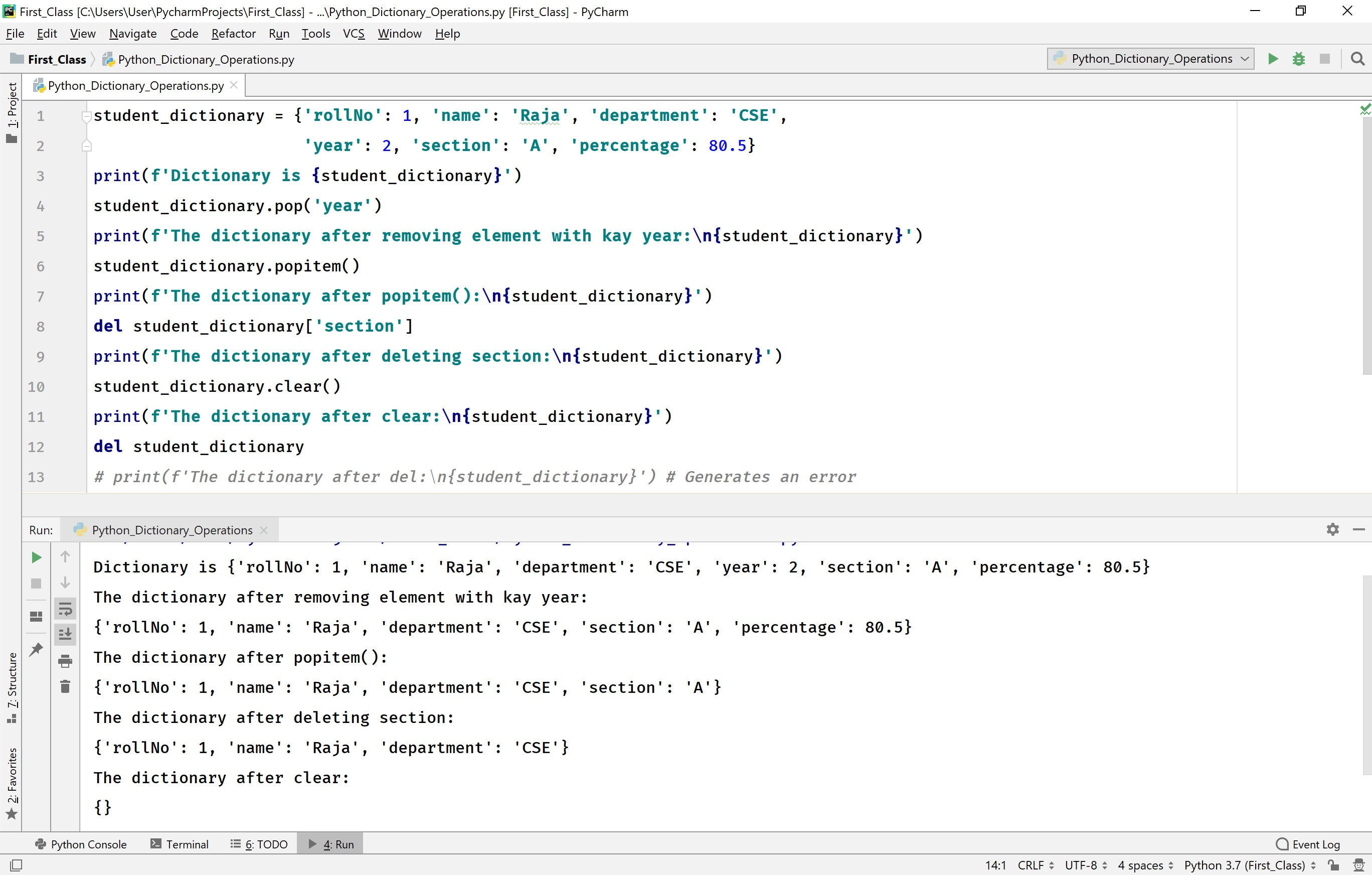The perfect place for easy learning...

×

# Python Dictionary

In Python, a dictionary is a collection of elements where each element is a pair of key and value. In Python, the dictionary data type (data structure) has implemented with a class known as dict. All the elements of a dictionary must be enclosed in curly braces, each element must be separated with a comma symbol, and every pair of key and value must be separated with colon ( : ) symbol.

## Creating a dictionary in Python

The general syntax for creating a dictionary in Python is as follows.

### Syntax

`dictionary_name = {key_1: value_1, key_2: value_2, key_3: value_3, ...}`

For example, consider the following code for creating a dictionary which stores the details of a student.

# Python code to illustrate creating a dictionary

``````
student_dictionary = {'rollNo': 1, 'name': 'Raja', 'department': 'CSE', 'year': 2}
print(type(student_dictionary))
```
```

When we run the above code, it produces the output as follows.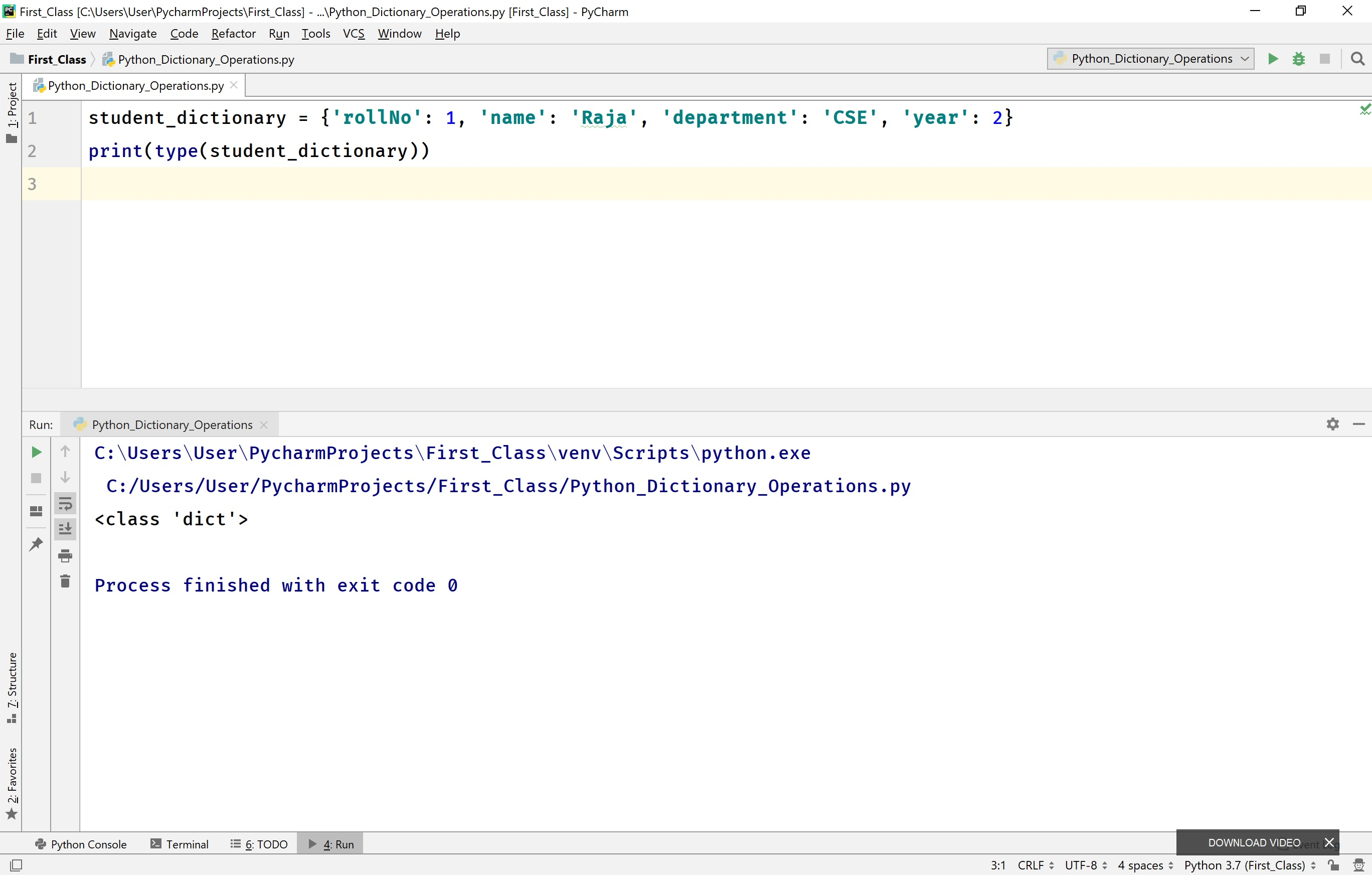In Python, a dictionary can also be created using the dictionary( ) constructor. The dictionary() constructor takes only one argument.

### Syntax

`dictionary_name = dict({key_1: value_1, key_2: value_2, key_3: value_3, ...})`

For example, consider the following code for creating a dictionary using dictionary() constructor which stores the details of a college.

# Python code to illustrate creating a dictionary using dict() constructor.

``````
student_dictionary = dict({'rollNo': 1, 'name': 'Raja', 'department': 'CSE', 'year': 2})
print(type(student_dictionary))
```
```

When we run the above code, it produces the output as follows.## Accessing Elements of a dictionary in Python

In Python, the dictionary elements are organized based on the keys. So, we can access using the key of a value in the dictionary. The Python provides the following ways to access the elements of a dictionary.

• Using Key as index - The elements of a dictionary can be accessed using the key as an index.

• # Example

````print(student_dictionary[name])`
```
• get( key ) - This method returns the value associated with the given key in the dictionary.

• # Example

````print(student_dictionary.get('name'))`
```
• Accessing the whole dictionary - In Python, we use the name of the dictionary to access the whole dictionary.

• # Example

````print(student_dictionary)`
```
• items( ) - This is a built-in method used to access all the elements of a dictionary in the form of a list of key-value pair.

• # Example

````print(student_dictionary.items())`
```
• keys( ) - This is a built-in method used to access all the keys in a dictionary in the form of a list.

• # Example

````print(student_dictionary.keys())`
```
• values( ) - This is a built-in method used to access all the values in a dictionary in the form of a list.

• # Example

````print(student_dictionary.values())`
```

For example, consider the following code for accessing the elements of a dictionary.

# Python code to illustrate accessing elements in a dictionary.

``````
student_dictionary = {'rollNo': 1, 'name': 'Raja', 'department': 'CSE', 'year': 2}
print(type(student_dictionary))
print(student_dictionary['rollNo']) # Accessing using key as index
print(student_dictionary.get('name')) # Accessing using get() method
print(student_dictionary) # Accessing whole dictionary using the name of the dictionary
print(student_dictionary.items()) # Accessing all elements of a dictionary using items() method
print(student_dictionary.keys()) # Accessing all keys in a dictionary using keys() method
print(student_dictionary.values()) # Accessing all value in a dictionary using values() method
```
```

When we run the above code, it produces the output as follows.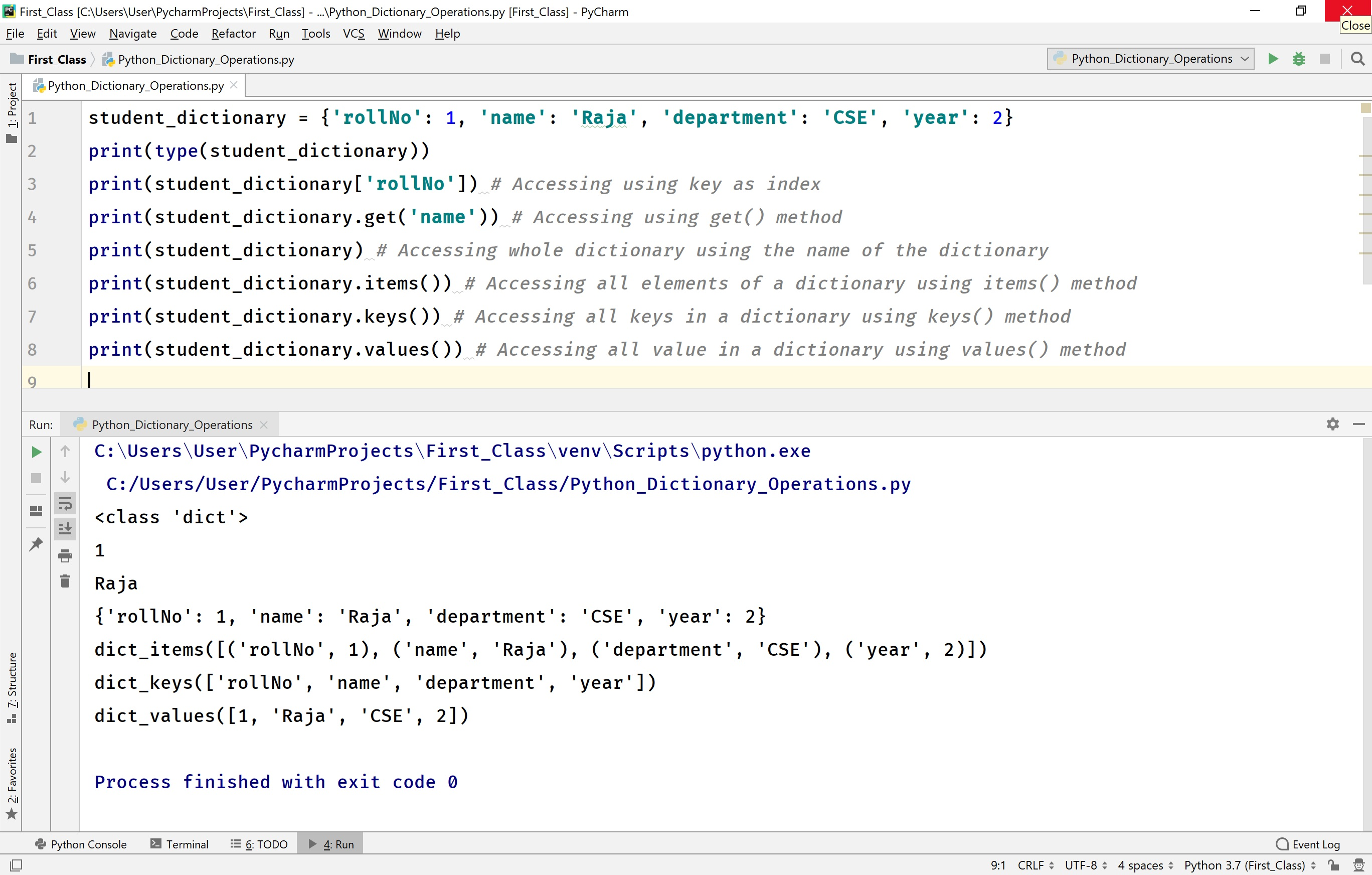## Changing an Element of a dictionary in Python

In Python, the value of a specific element in a dictionary can be changed using the respective key as an index. The following example shows changing the name to 'Seetha'.

# Python code to illustrate loop through a set.

``````
student_dictionary = {'rollNo': 1, 'name': 'Raja', 'department': 'CSE', 'year': 2}
print(f'Dictionary is {student_dictionary}')
student_dictionary['name'] = 'Seetha'
print(f'Dictionary after changing name to Seetha is - {student_dictionary}')
```
```

When we run the above code, it produces the output as follows.## Looping through a dictionary in Python

In Python, we can loop through a dictionary using for statement with a membership operator in.

For example, consider the following code to loop through a dictionary.

# Python code to illustrate loop through a dictionary.

``````
student_dictionary = {'rollNo': 1, 'name': 'Raja', 'department': 'CSE', 'year': 2}
print(f'Dictionary is {student_dictionary}')
for every_key in student_dictionary:
print(f'{every_key} --> {student_dictionary[every_key]}')
```
```

When we run the above code, it produces the output as follows.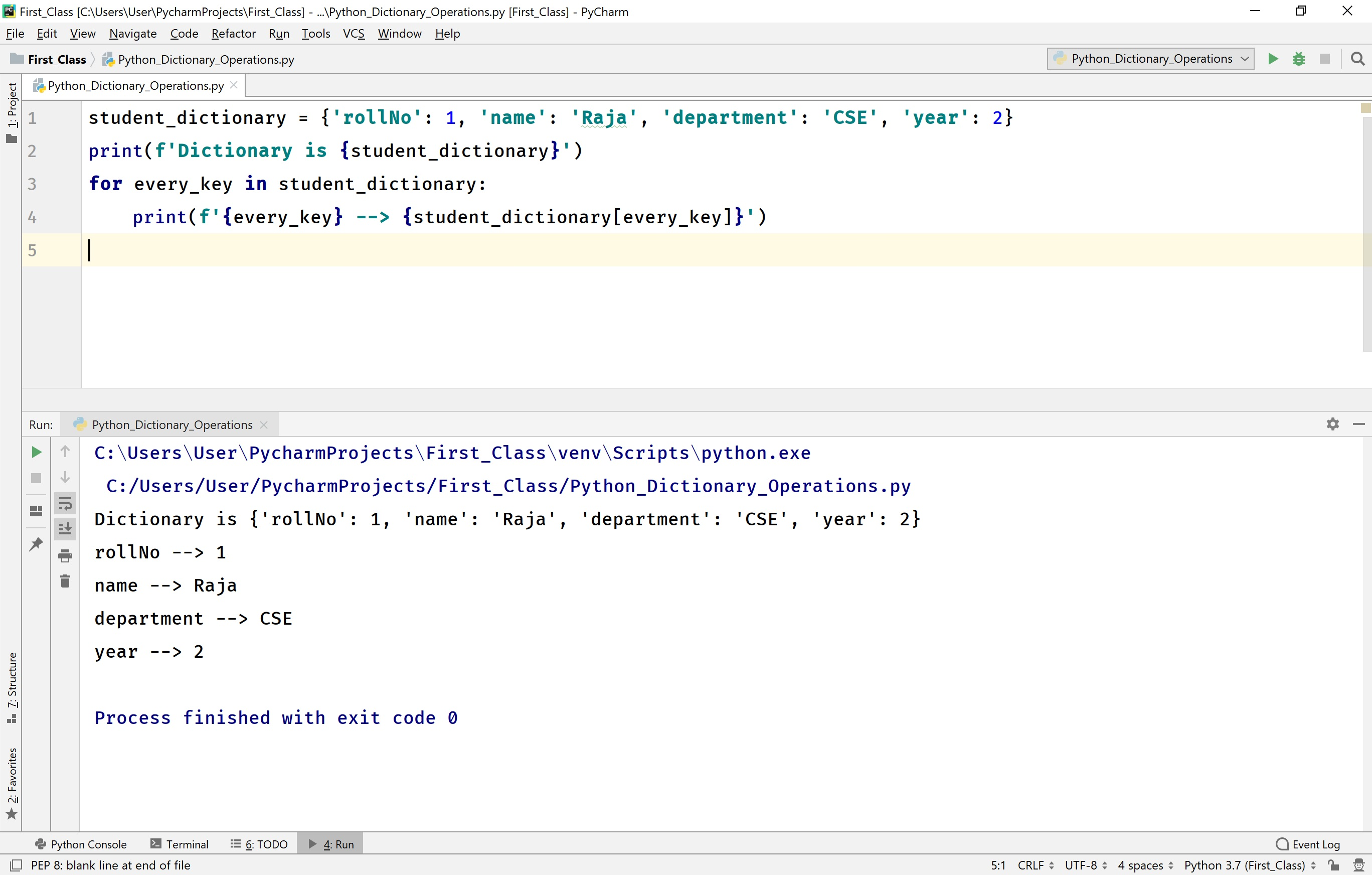## Existence of an element in a dictionary in Python

In Python, we can test whether an element is present in a dictionary or not using a membership operator 'in'.

For example, consider the following code to test the existence of an element in a dictionary.

# Python code to illustrate the existence of an element in a dictionary.

``````
student_dictionary = {'rollNo': 1, 'name': 'Raja', 'department': 'CSE', 'year': 2}
print(f'Dictionary is {student_dictionary}')
if 'Raja' in student_dictionary.values():
print(f'the value Raja is found in the dictionary {student_dictionary}')
else:
print(f'the value Raja is not found in the dictionary {student_dictionary}')
```
```

When we run the above code, it produces the output as follows.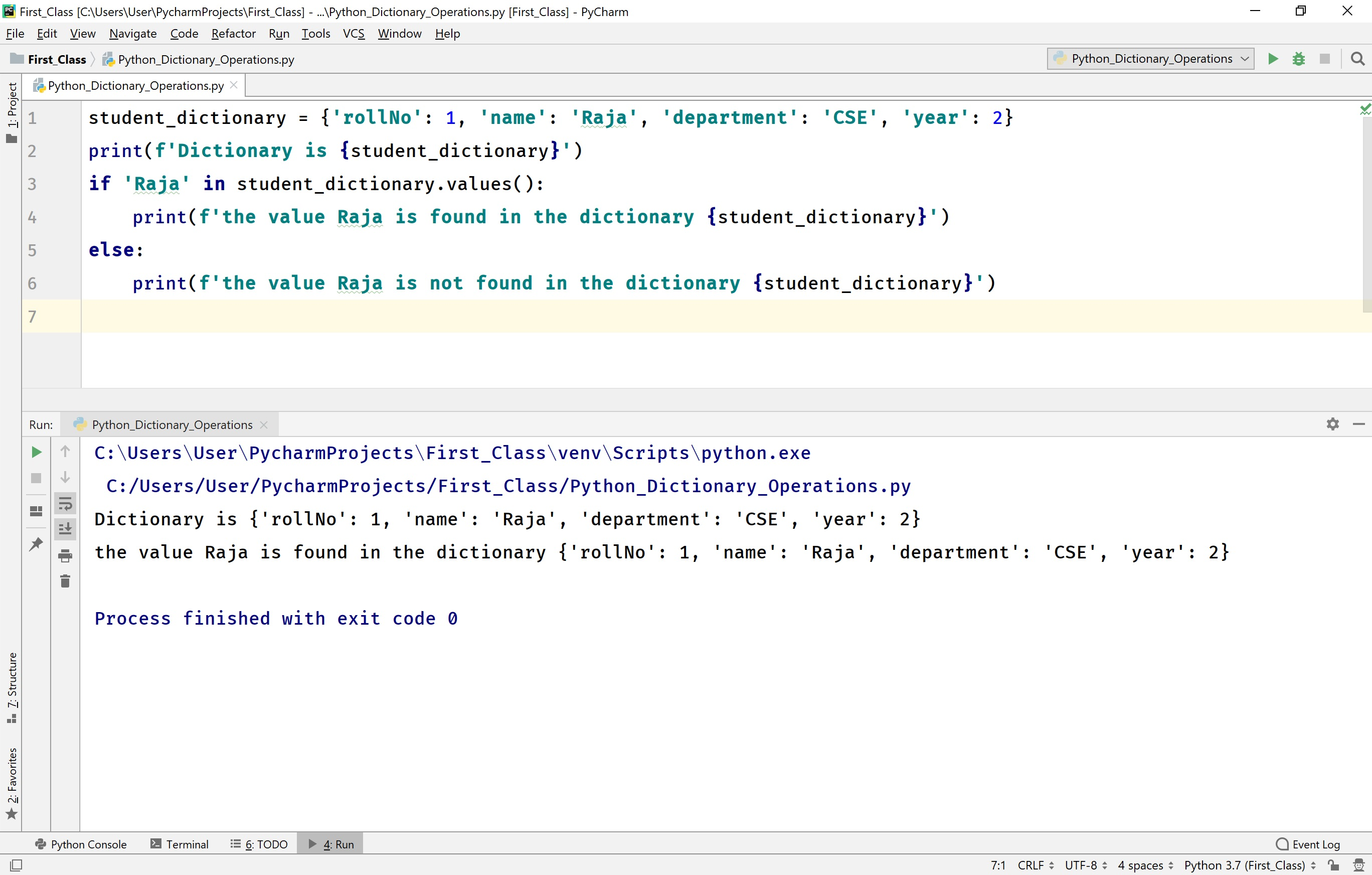## Finding the length of a dictionary in Python

The Python provides a built-in function len( ) to find the length of a dictionary. Here, the length of a dictionary is the total number of elements in that dictionary.

For example, consider the following code to find the length of a dictionary.

# Python code to illustrate the length of a dictionary.

``````
student_dictionary = {'rollNo': 1, 'name': 'Raja', 'department': 'CSE', 'year': 2}
print(f'Dictionary is {student_dictionary}')
print(f'Length of the dictionary is {len(student_dictionary)}')
```
```

When we run the above code, it produces the output as follows.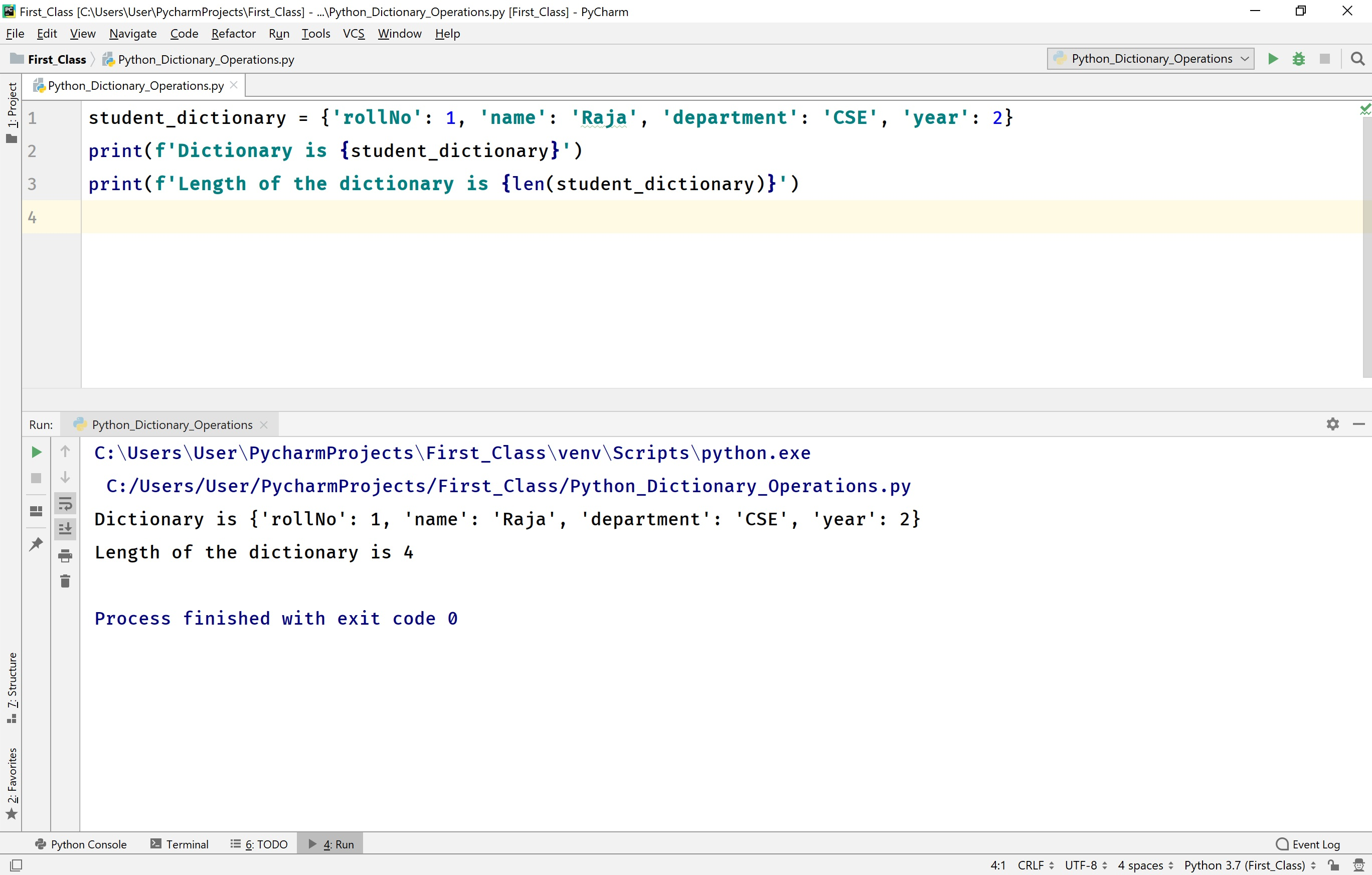## Adding an element to an existing dictionary in Python

Adding a new element to the existing dictionary is done by using a new key index and assigning a value to it.

Adding a new element to the existing dictionary is also performed using a built-in method update( key, value )

For example, consider the following code.

# Python code to illustrate adding an element to a dictionary.

``````
student_dictionary = {'rollNo': 1, 'name': 'Raja', 'department': 'CSE', 'year': 2}
print(f'Dictionary is {student_dictionary}')
student_dictionary['section'] = 'A' # Adding using new key assigned with a value
print(f'Dictionary after adding new element is\n{student_dictionary}')

student_dictionary.update({'percentage': 89.5}) # Adding using update() method
print(f'Dictionary after updated with new element is\n{student_dictionary}')
```
```

When we run the above code, it produces the output as follows.## Removing elements from a dictionary in Python

In Python, removing an element from the existing dictionary is performed using the following built-in methods.

• pop( key ) - This method removes the element with a specified key from the dictionary.

• popitem( ) - This method removes the last element from the dictionary.

• clear( ) - This method removes all the elements from the dictionary. That means the clear( ) method make the dictionary empty. This method returns the None value.

• del keyword with dict[key] - This keyword deletes the element with the specified key from the dictionary. Once the del keyword has used on a dictionary, we can not access it in the rest of the code.

• del keyword - This keyword deletes the dictionary completely. Once the del keyword has used on a dictionary, we can not access it in the rest of the code.

For example, consider the following code.

# Python code to illustrate remove operations in a dictionary.

``````
student_dictionary = {'rollNo': 1, 'name': 'Raja', 'department': 'CSE',
'year': 2, 'section': 'A', 'percentage': 80.5}
print(f'Dictionary is {student_dictionary}')
student_dictionary.pop('year')
print(f'The dictionary after removing element with kay year:\n{student_dictionary}')
student_dictionary.popitem()
print(f'The dictionary after popitem():\n{student_dictionary}')
del student_dictionary['section']
print(f'The dictionary after deleting section:\n{student_dictionary}')
student_dictionary.clear()
print(f'The dictionary after clear:\n{student_dictionary}')
del student_dictionary
# print(f'The dictionary after del:\n{student_dictionary}') # Generates an error
```
```

When we run the above code, it produces the output as follows.